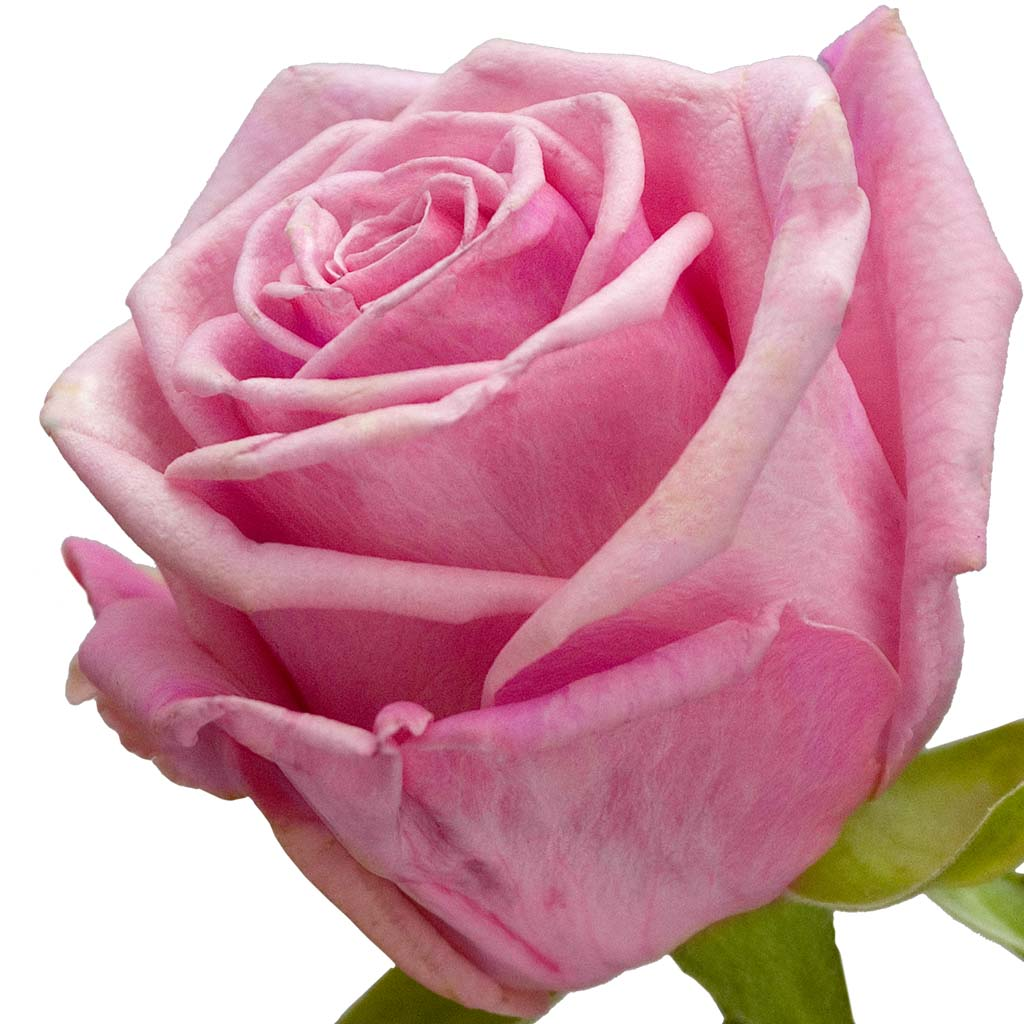# the andrea roseTo my darling sweet Andrea I miss you terribly my angel x x x x x x x x x x x x x x x x x x x x x x x x x x x x x x x x x x x x x x x x x x x x x x x x x x x x x x x x x x x x x x x x x x x x x x x x x x x x x x x x x x x x x x x x x x x x

The andrea rose was planted in our memorial rose garden in 2006.